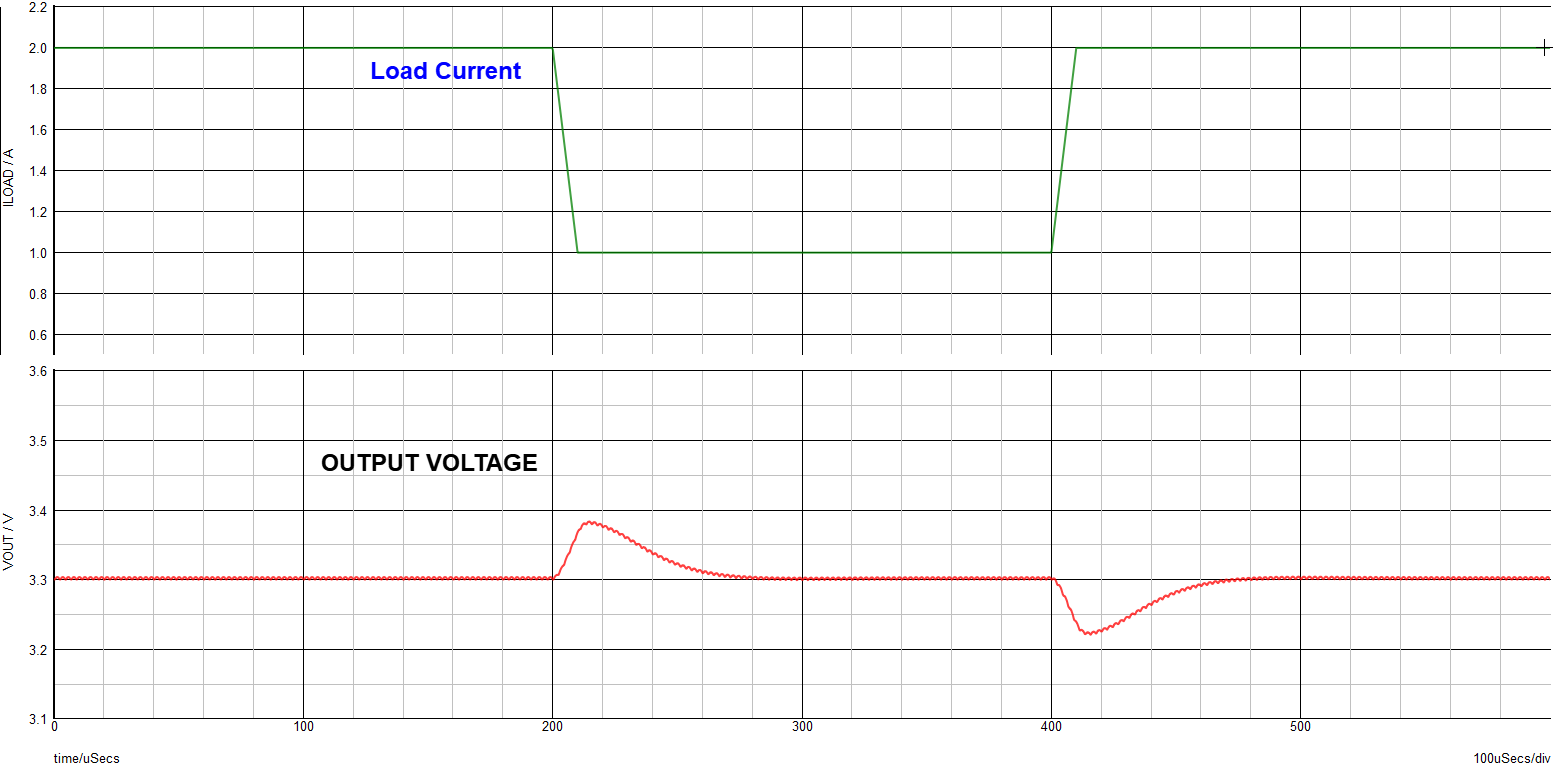# 利用外部调节来改善带集成补偿网络的降压稳压器瞬态性能## 快速回顾降压稳压器的峰值电流模式 (PCM) 控制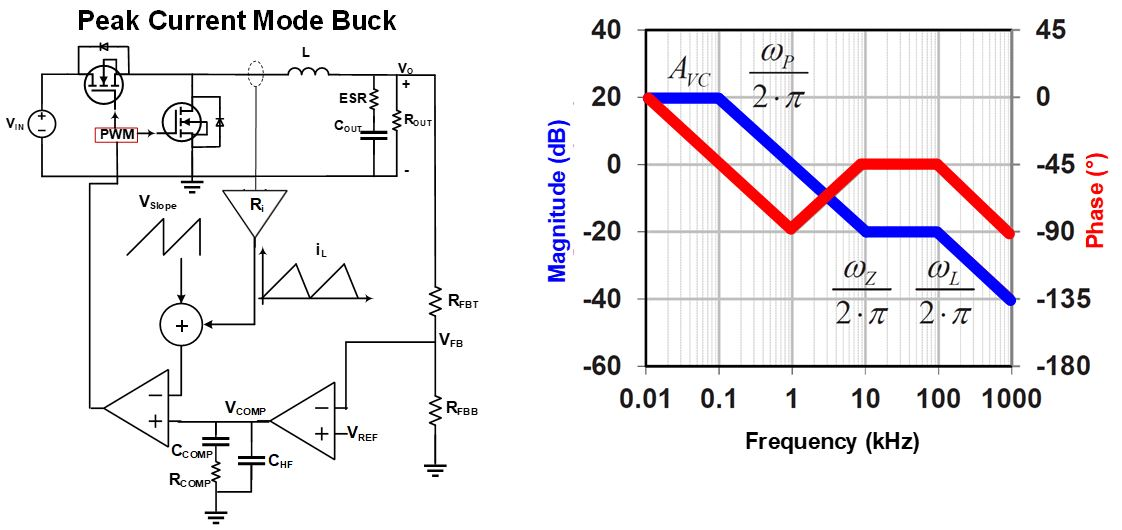$$\omega_{P} \approx \frac {1}{C_{OUT} \space x \space R_{OUT}}$$ $$\omega_L = {K_m \space x \space R_i \over L}$$

$$R_i = A \space x \space R{s}$$

$$K_m \approx {V_{IN} \over V_{SLOPE}}$$

$$\omega_Z = {1 \over C_{OUT} \space x \space ESR}$$

## 评估内部补偿网络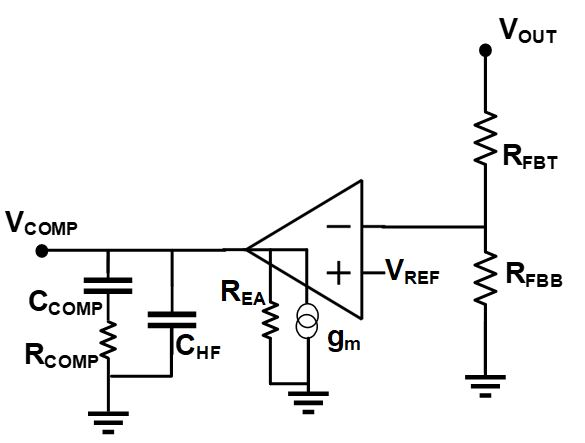II类补偿网络的零点频率和极点频率可以分别用公式 (6) 和公式 (7) 来计算：

$$\omega_{COMP}-Z1 = {1 \over R_{COMP} \space x \space C_{COMP}}$$ $$\omega_{COMP}-P1 = {1 \over R_{COMP} \space x \space C_{HF}}$$

$$BW = 0.1 \space x \space f_{SW}$$

$$0.1 \space x \space BW < f_{COMP-Z1}$$

$$f_{COMP-P1} = f_{SW} / 2$$

$$C_{HF} < 4\% \space x \space C_{COMP}$$

## 增加外部调节以进一步优化内部补偿网络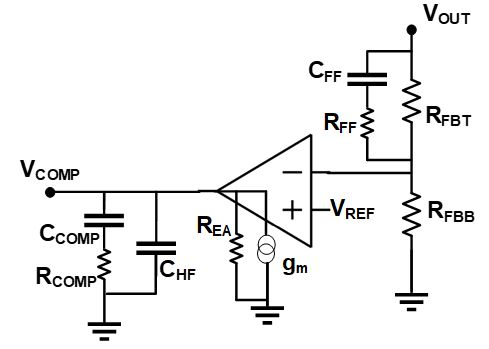CFF创建的零点频率可以用公式 (12) 来计算：

$$\omega_{COMP}-Z2 \approx {1 \over R_{FBT} \space x \space C_{FF}}$$

$$\omega_{COMP}-P2 \approx {1 \over R_{FF} \space x \space C_{FF}}$$

## 优化 PCM 降压稳压器中补偿网络的系统性方法

1. 将稳压器目标带宽设置为 0.1 x fSW.
2. 用公式（6）计算内部 COMP-Z1 频率，并确保它满足公式（14）设定的目标：
3. $$0.1 \space x \space BW < f_{COMP-Z1} < 0.2 \space x \space BW$$
4. 确保满足公式 (15) 设定的要求：
5. $$C_{HF} < 4\% \space x \space C_{COMP}$$
6. 一旦完成功率级设计，即运行初始波特图。确保：
• BW 接近目标值 (0.1 x fSW).
• 相位在目标 BW 处开始下降，而不是在此之前。
7. 如果步骤 4 中测得的 BW 未能接近步骤 1 中设定的目标 BW，则调整 FB 串联电阻，使 BW 与目标值一致。
8. 重新运行波特图并检查 PM ，确保FB 串联电阻调整之后，PM超过 60°。如果已达到，则忽略以下步骤；如果未达到，请继续执行步骤 7 到 10。
9. 设置CFF ，使 COMP-Z2 频率接近用公式 (16) 算出的值：
10. $$0.2 \space x \space (target\space BW) < f_{COMP-Z2} < 0.4 \space x \space (target\space BW)$$

1. 调整/增加 FB 串联电阻，使 BW 回到其原始目标值，因为在上一步中CFF 改变了幅度曲线。
2. 目标 BW（0.1 x fSW）和目标PM（>60°）应该已实现。
3. 可选步骤：为了在较高频率 (HF) 下获得更高的衰减，添加一个与CFF串联的电阻器 (RFF)（参见图 3），以生成第二个极点 (COMP-P2)。要正确设置 COMP-P2 频率 (fCOMP-P2)，请使用公式 (5) 和 fSW/2估算最小ESR零点频率，然后将两者中的较低者设置为 COMP-P2 频率目标值。调节RFF的值，使 COMP-P2 达到其目标值。注意，由该极点产生的负相位将在 0.1 x fCOMP-P2处生效，并且可能会略微降低 PM。

## 案例研究- MPQ4420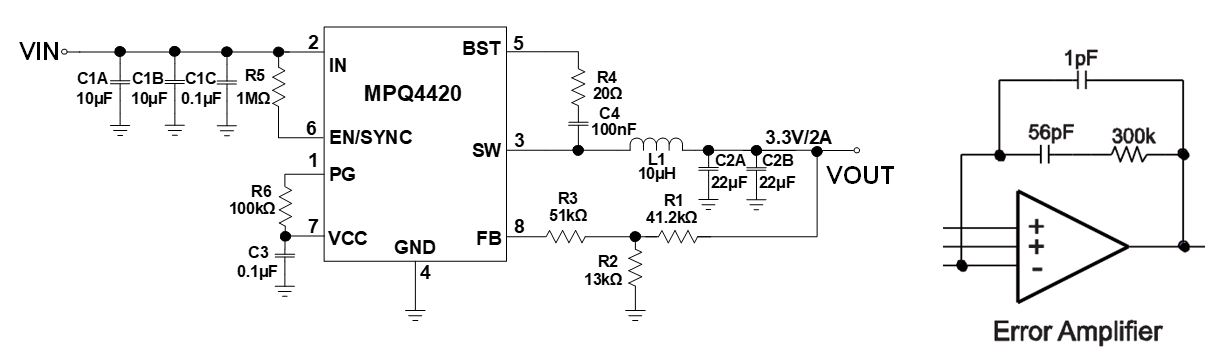1. MPQ4420开关频率为 400kHz，将目标带宽设置为该值的 10%，即 40kHz。
2. 用公式 (6) 计算 COMP-Z1 的频率，其结果约为9.5kHz。这与目标 COMP-Z 足够接近，因为根据公式 (14)，COMP-Z应介于 4kHz 和 8kHz 之间。
3. 比较CHF和CCOMP。根据图 4可知，CHF和CCOMP分别为 1pF 和 56pF。因此，CHF约为CCOMP的 2%。这满足公式（15）设定的要求。
4. 模拟上述原理图的波特图（包括功率级组件）。图 5(a) 和表 1显示了波特测量结果。其带宽为 72kHz，超过了40kHz的目标。而相位在 40kHz 左右开始下降，也符合目标预期。
5. 增大FB串联电阻。图 4 中的 R3 为FB 串联电阻，其典型值介于 10kΩ 和 100kΩ 之间。以 5kΩ 为步长逐步增大FB 串联电阻值，直到实现目标BW。在本例中，目标带宽由15kΩ FB 串联电阻实现。
6. 将第 5 步实现的 PM 与目标 PM 进行比较，目标 PM 应超过 60°。如表 1 所示，此时PM 仅为 34°。因此，需要额外的相位提升来实现目标 PM。
7. 添加第二个补偿器 (COMP-Z2) 以在 BW 频率处提供额外的相位提升。公式 (12) 中算出的CFF值为220pF。图 5(b) 显示了在系统中添加额外相位提升后的波特测量结果。可以看到最大相位提升发生在40kHz的目标带宽附近。
8. 更改/增加 FB 串联电阻以将 BW返回到其目标值 (40kHz)，因为添加CFF/COMP-Z2 会将 BW 增加到 104kHz。图 5(c) 显示了波特测量结果。
9. 确保系统以 40kHz (0.1 x fSW) 的带宽和超过 60° 的 PM 实现优化。这两个目标应该都已实现。
10. 为使系统在高于开关频率时获得更高衰减，添加RFF，与CFF串联，以形成第二个极点 (COMP-P2)。由于开关频率为 400kHz < 1MHz，因此将fSW /2设置为定位COMP-P2 的目标频率。已知目标fCOMP-P2和CFF，则RFF的初始值应为 3.6kΩ。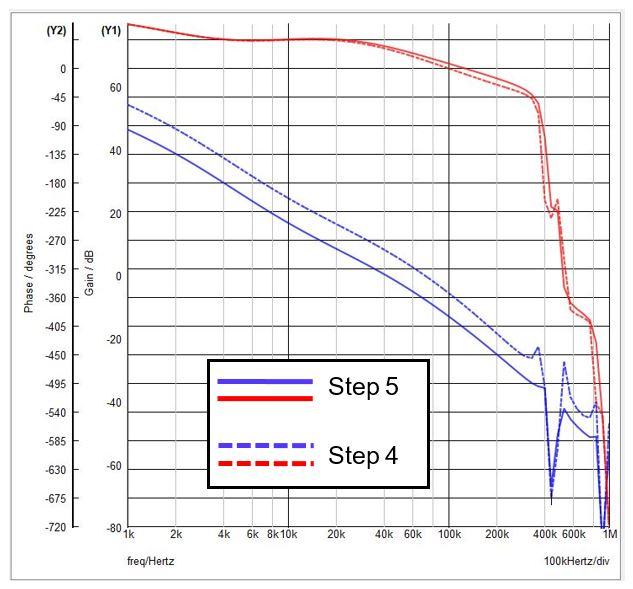a) 步骤4 至步骤 5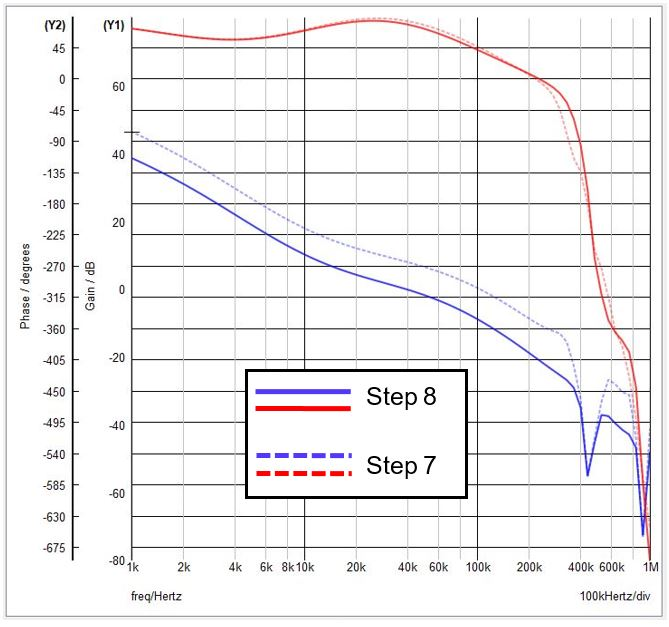b) 步骤5 至步骤 7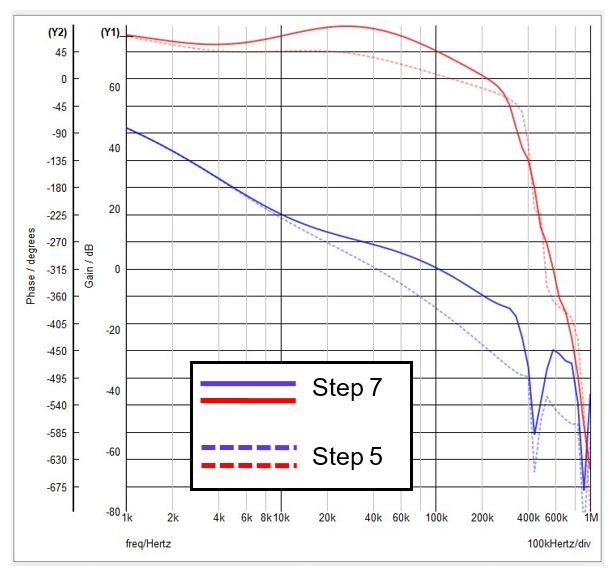c) 步骤7 至步骤 8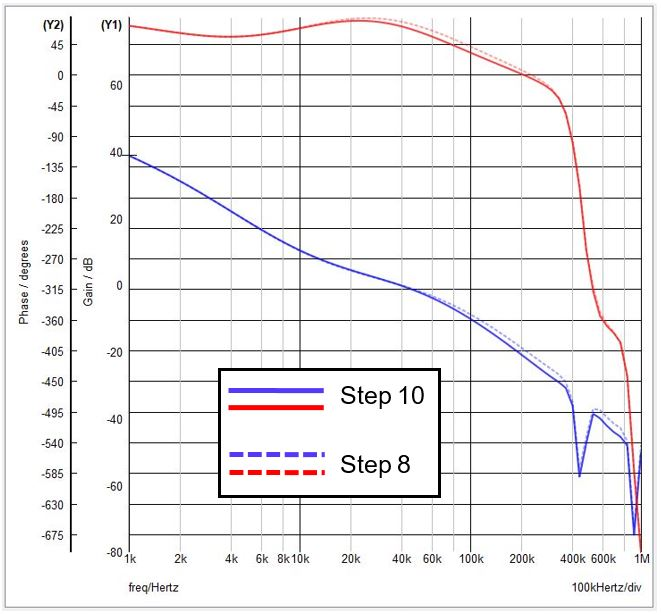d) 步骤 8 至步骤 10

 步骤 4 步骤 5 步骤 7 步骤 8 步骤 10 FB 串联电阻 0Ω 15kΩ 15kΩ 51kΩ 51kΩ CFF NP NP 220pF 220pF 220pF RFF NP NP NP NP NP BW 72kHz 41kHz 104kHz 40kHz 38kHz PM 12º 34º 44º 78º 72º

## MPQ4420 瞬态性能验证